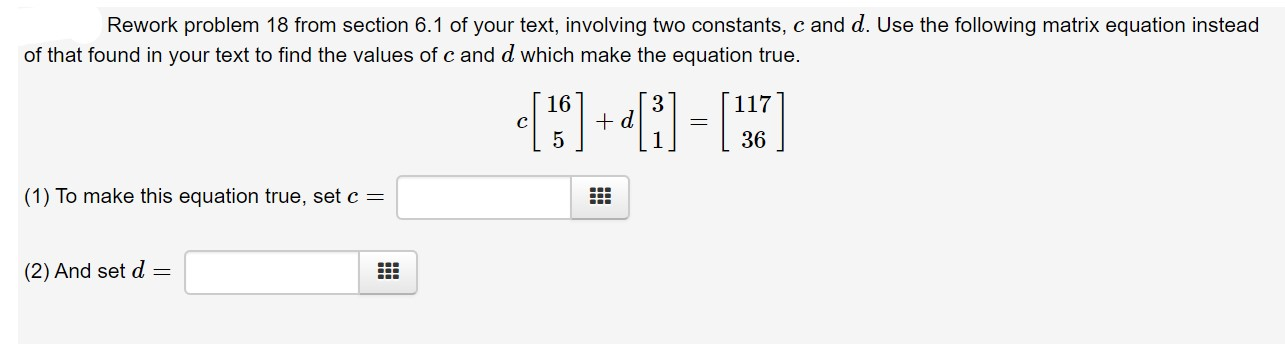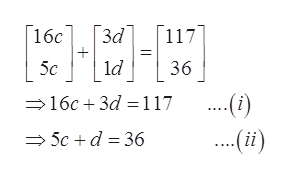# Rework problem 18 from section 6.1 of your text, involving two constants, c and d. Use the following matrix equation insteadof that found in your text to find the values of c and d which make the equation true16d117C36(1) To make this equation true, set c(2) And set d =

Questionhelp_outlineImage TranscriptioncloseRework problem 18 from section 6.1 of your text, involving two constants, c and d. Use the following matrix equation instead of that found in your text to find the values of c and d which make the equation true 16 d 117 C 36 (1) To make this equation true, set c (2) And set d = fullscreen
check_circleExpert Solution
Step 1

Given

Step 2

Solvehelp_outlineImage Transcriptionclose16c Гза 117 ld 5c 36 16c 3d= 1 17 ..(i) ..(i) 5c+d 36 fullscreen
Step 3

Solve equation (i) and equation (ii) and get the value of c and d

for that...

### Want to see the full answer?

See Solution

#### Want to see this answer and more?

Solutions are written by subject experts who are available 24/7. Questions are typically answered within 1 hour*

See Solution
*Response times may vary by subject and question
Tagged in

### Math